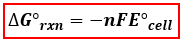# Problem: Consider a fuel cell that uses the reaction of ethanol with oxygen to produce electricity, CH3CH2OH(l) + 3O2(g) → 2CO2(g)+ 3H2O(l)Determine the E°cell for this cell at 25°.E°cell = v

###### FREE Expert Solution
93% (484 ratings)
###### FREE Expert Solution

We’re asked to calculate the cell potential (E°cell)­ of a fuel cell. The cell potential of an electrochemical cell is related to Gibb's free energy of the reaction by the following equation:∆G° = Gibb’s Free Energy
n = number of electrons transferred
= Faraday’s constant = 96485 C/(mol e-)
cell =standard cell potential

We will solve the problem in the following steps:

1. Calculate ΔG˚rxn (since it is not given)
2. Determine the number of electrons involved in the reaction by balancing the redox reaction
3. Calculate E˚cell using the equation above.

93% (484 ratings)###### Problem Details

Consider a fuel cell that uses the reaction of ethanol with oxygen to produce electricity,

CH3CH2OH(l) + 3O2(g) → 2CO2(g)+ 3H2O(l)

Determine the E°cell for this cell at 25°.

cell = v

What scientific concept do you need to know in order to solve this problem?

Our tutors have indicated that to solve this problem you will need to apply the Cell Potential concept. If you need more Cell Potential practice, you can also practice Cell Potential practice problems.

What is the difficulty of this problem?

Our tutors rated the difficulty ofConsider a fuel cell that uses the reaction of ethanol with ...as medium difficulty.

How long does this problem take to solve?

Our expert Chemistry tutor, Sabrina took 13 minutes and 15 seconds to solve this problem. You can follow their steps in the video explanation above.

What professor is this problem relevant for?

Based on our data, we think this problem is relevant for Professor Madison's class at PITT.